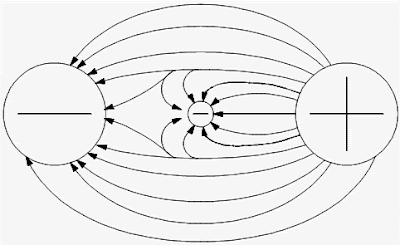## Monday, 24 September 2018

### Electric Potential and Potential DifferencePotential difference is the term used to describe how large the electrostatic force is between two charged objects. If a charged body is placed between two objects with a potential difference, the charged body will try to move in one direction, depending upon the polarity of the object.

If an electron is placed between a negatively-charged body and a positively-charged body, the action due to the potential difference is to push the electron toward the positively-charged object.

The electron, being negatively charged, will be repelled from the negatively-charged object and attracted by the positively-charged object, as shown in Figure 1.Fig-1: potential difference between two charged objects

Due to the force of its electrostatic field, these electrical charges have the ability to do work by moving another charged particle by attraction and/or repulsion.

This ability to do work is called “potential”; therefore, if one charge is different from another, there is a potential difference between them. The sum of the potential differences of all charged particles in the electrostatic field is referred to as electromotive force (EMF).

The basic unit of measure of potential difference is the “volt“. The symbol for potential difference is “V” indicating the ability to do the work of forcing electrons to move.

Because the volt unit is used, potential difference is also called “voltage”

Voltage

The basic unit of measure for potential difference is the volt (symbol V), and, because the volt unit is used, potential difference is called voltage . An object’s electrical charge is determined by the number of electrons that the object has gained or lost. Because such a large number of electrons move, a unit called the “coulomb” is used to indicate the charge. One coulomb is equal to 6.28 x 1018 (billion, billion) electrons.

For example, if an object gains one coulomb of negative charge, it has gained 6,280,000,000,000,000,000 extra electrons. A volt is defined as a difference of potential causing one coulomb of current to do one joule of work.

A volt is also defined as that amount of force required to force one ampere of current through one ohm of resistance. The latter is the definition with which we will be most concerned in this module.

Source: Web based Article about Electric Potential